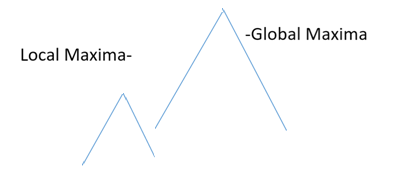# Hill Climbing Search in Artificial Intelligence

In this article, we will learn about the hill climbing in the heuristic search which is used in the field of AI for problem-solving using search techniques. We will learn about what the hill climbing search is and how it works, and what algorithm it follows? By Monika Sharma Last updated : April 12, 2023

## Hill Climbing Search

Hill climbing is a variety of Depth-First search. In this type of search (heuristic search), feedback is used to decide the next move in the state space. It is basically used for mathematical computations in the field of Artificial Intelligence.

## Concept of Hill Climbing

The main concept of hill climbing can be understood as follows:

suppose we are climbing the hill and are present at some point. Our task is to reach the peak point of the hill. So, we look at the neighboring points where we can step. If that step takes us closer towards the peak, then we take that step, but if it takes us farther, then we do not take it. If more than two options are available to choose from, then we choose the best one which brings us closest to our goal state.

So, the strategy that we follow in the hill-climbing algorithm is that we look at the neighboring point, if it is at a level which is higher than our current position, then we take that step, else we do a further comparison of the other neighboring points.

## Hill Climbing Search Algorithm

The following are the steps of hill climbing search algorithm:

1. Step 1: Evaluate the current initial state. If it is the goal state, then return and quit.
2. Step 2: If the current state is not the goal state, then loop until a solution is found or there are no further operations left for comparison.
3. Step 3: Select a new state for comparison.
4. Step 4: Evaluate the new state:
• If it is the goal state, i.e. the highest peak, then quit.
• If it is better than the current state, then make it the new current state.
• If it is not better than the current state, then go to step 2.

## Limitations (Problems) That Occur in Hill Climbing Search

The following are the limitations (problems) that occur in hill climbing search:

### 1. Local Maxima

It is a state which is better than all the existing neighboring states but is not the peak point of the hill.### 2. Plateau

A plateau is a flat area and therefore no neighboring state in the search space exists so that it is better than the current point because all of them lie on the same plane.### 3. Ridge

It is an area in the search space which is higher than the surrounding areas but cannot be searched in a simple move.